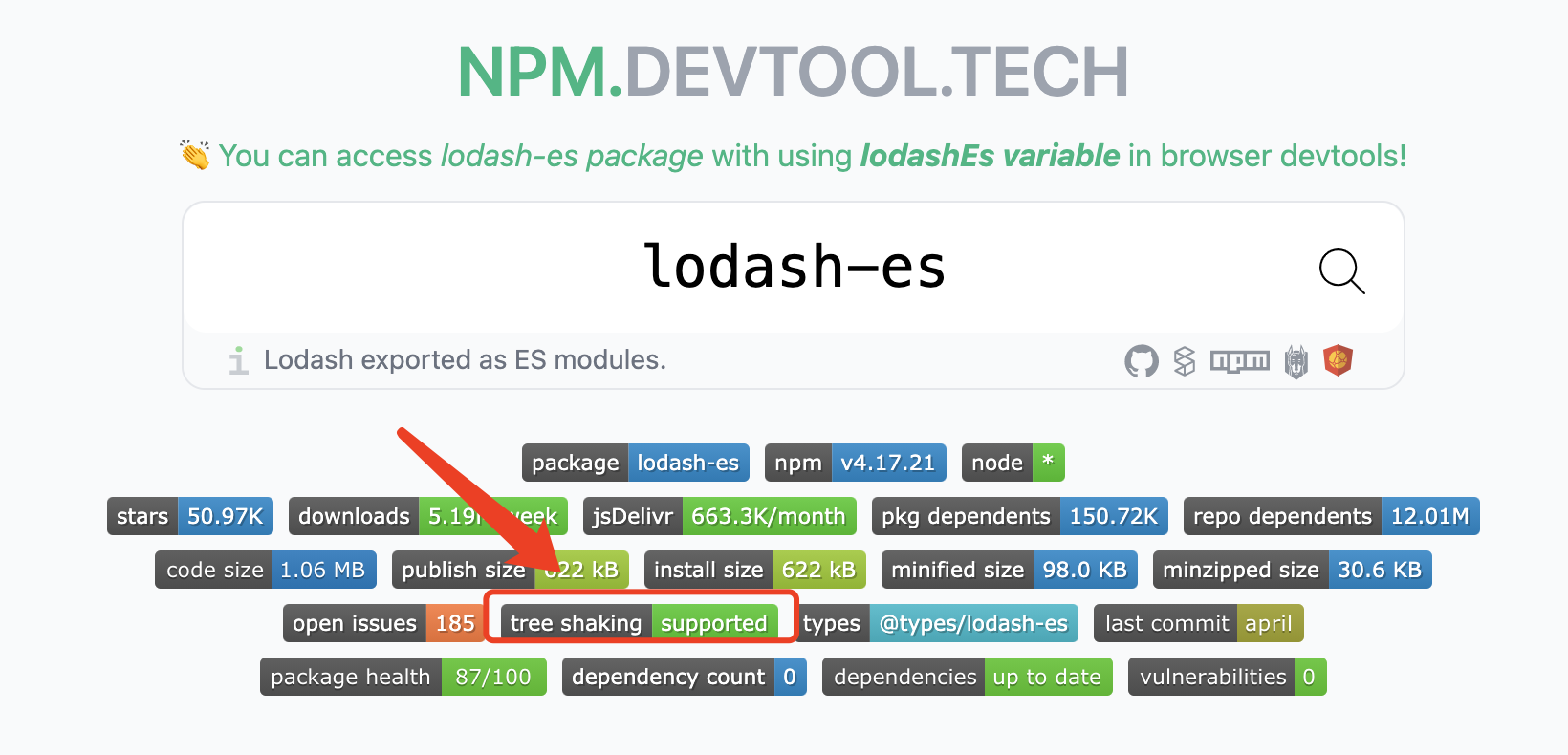# # Tree Shaking 的原理是什么

Issue

Author

Author

AST

Author

`Tree Shaking` 指基于 ES Module 进行静态分析，通过 AST 将用不到的函数进行移除，从而减小打包体积。

``````/* TREE-SHAKING */
import { sum } from "./maths.js";

console.log(sum(5, 5)); // 10
``````
``````// maths.js

export function sum(x, y) {
return x + y;
}

// 由于 sub 函数没有引用到，最终将不会对它进行打包
export function sub(x, y) {
return x - y;
}
``````

``````// maths.js

function sum(x, y) {
return x + y;
}

/* TREE-SHAKING */

console.log(sum(5, 5));
``````

## #`import *`

``````import * as maths from "./maths";

// Tree Shaking 依然生效
maths.sum(3, 4);
maths["sum"](3, 4);
``````

`import * as maths`，其中 `maths` 的数据结构是固定的，无复杂数据，通过 AST 分析可查知其引用关系。

``````const maths = {
sum() {},
sub() {},
};
``````

## # JSON TreeShaking

`Tree Shaking` 甚至可对 JSON 进行优化。原理是因为 JSON 格式简单，通过 AST 容易预测结果，不像 JS 对象有复杂的类型与副作用。

``````{
"a": 3,
"b": 4
}
``````
``````import obj from "./main.json";

// obj.b 由于未使用到，仍旧不会被打包
console.log(obj.a);
``````

## # 引入支持 Tree Shaking 的 PackageLast Updated: 11/27/2021, 6:11:48 PM